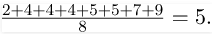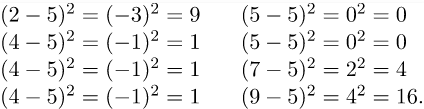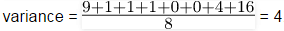# Mean, Probability Notes | Study GATE Computer Science Engineering(CSE) 2023 Mock Test Series - GATE

## GATE: Mean, Probability Notes | Study GATE Computer Science Engineering(CSE) 2023 Mock Test Series - GATE

The document Mean, Probability Notes | Study GATE Computer Science Engineering(CSE) 2023 Mock Test Series - GATE is a part of the GATE Course GATE Computer Science Engineering(CSE) 2023 Mock Test Series.
All you need of GATE at this link: GATE

Mean, Variance

Mean is average of a given set of data. Let us consider below example

2, 4, 4, 4, 5, 5, 7, 9

These eight data points have the mean (average) of 5:Variance is sum of squares of differences between all numbers and means.
Deviation for above example. First, calculate the deviations of each data point from the mean, and square the result of each:The document Mean, Probability Notes | Study GATE Computer Science Engineering(CSE) 2023 Mock Test Series - GATE is a part of the GATE Course GATE Computer Science Engineering(CSE) 2023 Mock Test Series.
All you need of GATE at this link: GATEUse Code STAYHOME200 and get INR 200 additional OFF

## GATE Computer Science Engineering(CSE) 2023 Mock Test Series

136 docs|165 tests

Track your progress, build streaks, highlight & save important lessons and more!

,

,

,

,

,

,

,

,

,

,

,

,

,

,

,

,

,

,

,

,

,

,

,

,

;